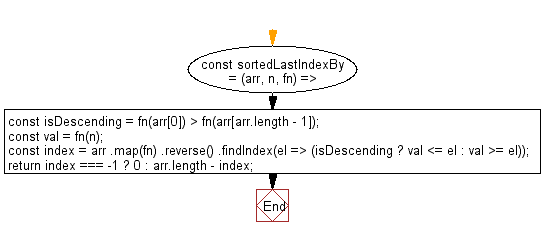# JavaScript : Get the highest index at which value should be inserted into array in order to maintain its sort order, based on a provided iterator function

## JavaScript: Exercise-140 with Solution

Write a JavaScript program to get the highest index at which value should be inserted into array in order to maintain its sort order, based on a provided iterator function.

• Loosely check if the array is sorted in descending order.
• Use Array.prototype.map() to apply the iterator function to all elements of the array.
• Use Array.prototype.reverse() and Array.prototype.findIndex() to find the appropriate last index where the element should be inserted, based on the provided iterator function.

Sample Solution:

JavaScript Code:

``````//#Source https://bit.ly/2neWfJ2
const sortedLastIndexBy = (arr, n, fn) => {
const isDescending = fn(arr) > fn(arr[arr.length - 1]);
const val = fn(n);
const index = arr
.map(fn)
.reverse()
.findIndex(el => (isDescending ? val <= el : val >= el));
return index === -1 ? 0 : arr.length - index;
};
console.log(sortedLastIndexBy([{ x: 4 }, { x: 5 }], { x: 4 }, o => o.x));
```
```

Sample Output:

```1
```

Flowchart:Live Demo:

See the Pen javascript-basic-exercise-140-1 by w3resource (@w3resource) on CodePen.

Improve this sample solution and post your code through Disqus

What is the difficulty level of this exercise?

Test your Programming skills with w3resource's quiz.

﻿

## JavaScript: Tips of the Day

Returns an array of n-tuples of consecutive elements

Example:

```const tips_arr = (n, arr) =>  n > arr.length ? [] : arr.slice(n - 1).map((v, i) =>[...arr.slice(i, i + n - 1), v]);
console.log(tips_arr(2, [1, 2, 3, 4, 5]));
console.log(tips_arr(3, [1, 2, 3, 4, 5]));
console.log(tips_arr(5, [1, 2, 3, 4]));
```

Output:

```[[1, 2], [2, 3], [3, 4], [4, 5]]
[[1, 2, 3], [2, 3, 4], [3, 4, 5]]
[]
```